# College Chemistry : Isotopes

## Example Questions

### Example Question #256 : College Chemistry

Isotopes differ in the number of __________.

covalent bonds

electrons

protons

neutrons

lone pairs

neutrons

Explanation:

By definition, isotopes of a given element have the same number of protons and electrons, but differ in the number of neutrons. This causes a difference in the mass number (protons + neutrons) as well. Neither the number of protons nor the number of electrons changes with different isotopes of the same element.

### Example Question #257 : College Chemistry

Which of the following is not an isotope of hydrogen?

Tritium

Protium

Deuterium

Quatrium

Quatrium

Explanation:

Isotopes are versions of an element with different numbers of neutrons. Hydrogen has three naturally occurring isotopes.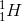, sometimes called protium, contains one electron, one proton, and no neutrons.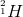, called deuterium, contains one electron, one proton, and one neutron.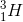, called tritium, contains one electron, one proton, and two neutrons. Hydrogen has no such isotope that contains three neutrons.

### Example Question #258 : College Chemistry

Chromium has four naturally occurring isotopes.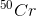has a mass of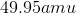and an abundance of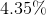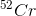has a mass of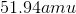and an abundance of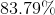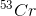has a mass of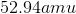and an abundance of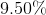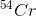has a mass of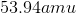and an abundance of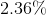.

Using this information, estimate the atomic mass of chromium, in atomic mass units.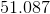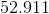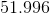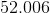Explanation:

In order to find the molar mass of an atom from its isotopes and their natural abundances, use the following equation: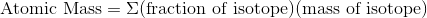for all the given isotopes.

Since chromium has four isotopes, we will write the following equation to find its atomic mass: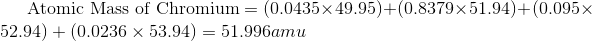### Example Question #259 : College Chemistry

Isotopes of a given element differ in the number of __________.

Electrons

Protons

Photons

Neutrons

Some elements, however, also have different "versions" of themselves: atoms which have a different number of neutrons, called isotopes. For example, there are three isotopes of hydrogen.has one proton and zero neutrons.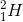has one proton and one neutron. Lastly,has one proton and two neutrons. Carbon is another such element that has different isotopes.